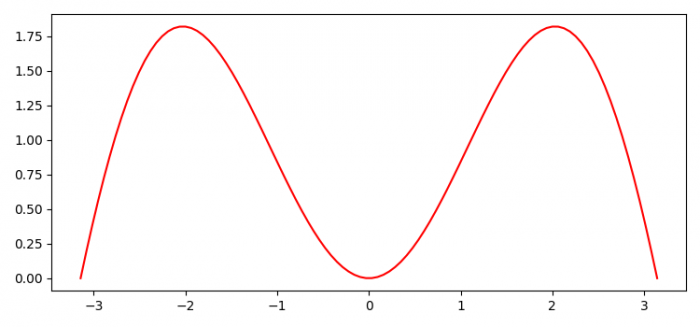# How to save an image with matplotlib.pyplot?

To save an image with matplotlib.pyplot.savefig(), we can take the following steps −

• Set the figure size and adjust the padding between and around the subplots.
• Create x and y data points using numpy.
• Plot x and y data points using plot() method.
• To save the figure, use savefig() method.

## Example

import numpy as np
import matplotlib.pyplot as plt

plt.rcParams["figure.figsize"] = [7.50, 3.50]
plt.rcParams["figure.autolayout"] = True

x = np.linspace(-np.pi, np.pi, 100)

plt.plot(x, np.sin(x) * x, c='red')

plt.savefig("myimage.png")

## Output

When we execute the code, it will save the following image as "myimage.png" in the Project directory.Updated on: 03-Aug-2021

4K+ Views